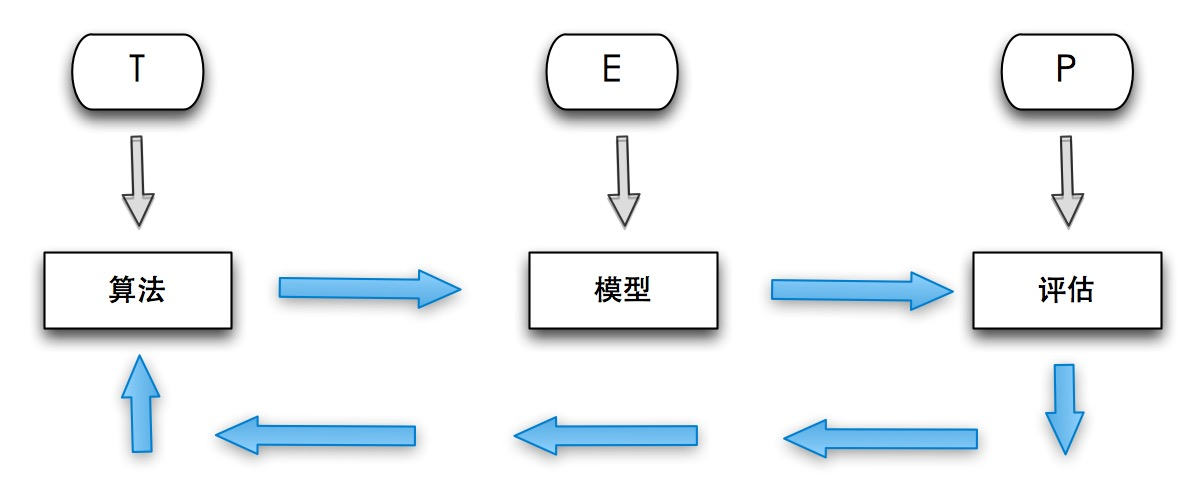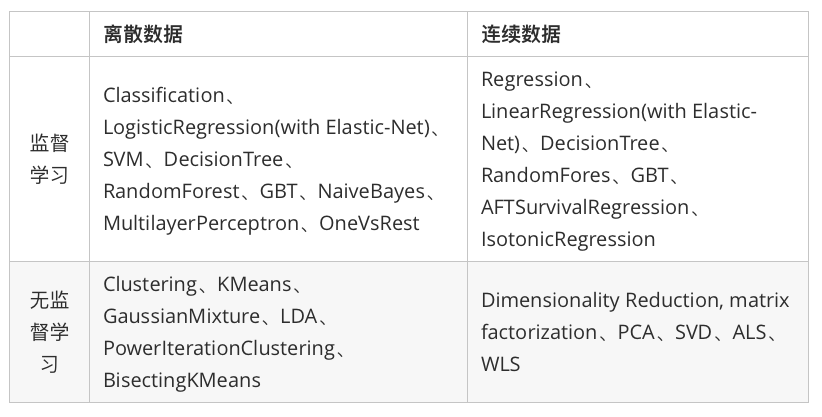#Spark MLlib

0
0
01. 云栖社区>
2. 博客>
3. 正文

## Spark MLlib

wsc449 2018-01-17 11:22:06 浏览682

### 1. 机器学习### 3. Spark 机器学习库MLLib

MLlib是Spark的机器学习（Machine Learning）库，旨在简化机器学习的工程实践工作，并方便扩展到更大规模。MLlib由一些通用的学习算法和工具组成，包括分类、回归、聚类、协同过滤、降维等，同时还包括底层的优化原语和高层的管道API。具体来说，其主要包括以下几方面的内容：

Spark 机器学习库从 1.2 版本以后被分为两个包：
spark.mllib包含基于RDD的原始算法API。Spark MLlib 历史比较长，在1.0 以前的版本即已经包含了，提供的算法实现都是基于原始的 RDD。
spark.ml 则提供了基于DataFrames 高层次的API，可以用来构建机器学习工作流（PipeLine）。ML Pipeline 弥补了原始 MLlib 库的不足，向用户提供了一个基于 DataFrame 的机器学习工作流式 API 套件。

Spark在机器学习方面的发展非常快，目前已经支持了主流的统计和机器学习算法。纵观所有基于分布式架构的开源机器学习库，MLlib可以算是计算效率最高的。MLlib目前支持4种常见的机器学习问题: 分类、回归、聚类和协同过滤。下表列出了目前MLlib支持的主要的机器学习算法：MLlib 是spark的可以扩展的机器学习库，由以下部分组成：通用的学习算法和工具类，包括分类，回归，聚类，协同过滤，降维，当然也包括调优的部分

• Data types
• Basic statistics (基本统计)
• summary statistics 概括统计
• correlations 相关性
• stratified sampling 分层取样
• hypothesis testing 假设检验
• random data generation 随机数生成
• Classification and regression (分类一般针对离散型数据而言的，回归是针对连续型数据的。本质上是一样的)
• linear models (SVMs, logistic regression, linear regression)线性模型（支持向量机，逻辑回归，线性回归）
• naive Bayes 贝叶斯算法
• decision trees 决策树
• ensembles of trees (Random Forests and Gradient-Boosted Trees)
多种树（随机森林和梯度增强树）
• Collaborative filtering 协同过滤
• alternating least squares (ALS) (交替最小二乘法(ALS) )
• Clustering 聚类
• k-means k均值算法
• Dimensionality reduction (降维)
• singular value decomposition (SVD) 奇异值分解
• principal component analysis (PCA) 主成分分析
• Feature extraction and transformation 特征提取和转化
• Optimization (developer) 优化部分
• stochastic gradient descent 随机梯度下降
• limited-memory BFGS (L-BFGS) 短时记忆的BFGS (拟牛顿法中的一种,解决非线性问题)

### 4. 线性回归实例

``````import org.apache.spark.SparkConf;
import org.apache.spark.api.java.JavaRDD;
import org.apache.spark.api.java.JavaSparkContext;
import org.apache.spark.api.java.function.Function;
import org.apache.spark.mllib.evaluation.RegressionMetrics;
import org.apache.spark.mllib.linalg.Vectors;
import org.apache.spark.mllib.regression.LabeledPoint;
import org.apache.spark.mllib.regression.LinearRegressionModel;
import org.apache.spark.mllib.regression.LinearRegressionWithSGD;

import scala.Tuple2;

public class SparkMLlibLinearRegression {

public static void main(String[] args) {

SparkConf conf = new SparkConf();
JavaSparkContext sc = new JavaSparkContext(args, "Spark", conf);

JavaRDD<String> data = sc.textFile(path);
JavaRDD<LabeledPoint> parsedData = data.map(new Function<String, LabeledPoint>() {
@Override
public LabeledPoint call(String line) throws Exception {
String[] parts = line.split(",");
String[] features = parts.split(" ");
double[] v = new double[features.length];
for (int i = 0; i < v.length; i++) {
v[i] =  Double.parseDouble(features[i]);
}
return new LabeledPoint(Double.parseDouble(parts), Vectors.dense(v));
}
});
parsedData.cache();

// Building the model
int numIterations = 100;
double stepSize = 0.00000001;
final LinearRegressionModel model =
LinearRegressionWithSGD.train(JavaRDD.toRDD(parsedData), numIterations, stepSize);

// Evaluate model on training examples and compute training error
JavaRDD<Tuple2<Double, Double>> valuesAndPreds = parsedData.map(new Function<LabeledPoint, Tuple2<Double, Double>>(){
@Override
public Tuple2<Double, Double> call(LabeledPoint point)
throws Exception {
double prediction = model.predict(point.features());
return new Tuple2<Double, Double>(prediction, point.label());
}

});

double MSE = new JavaDoubleRDD(valuesAndPreds.map(
new Function<Tuple2<Double, Double>, Object>() {
public Object call(Tuple2<Double, Double> pair) {
return Math.pow(pair._1() - pair._2(), 2.0);
}
}
).rdd()).mean();

System.out.println("training Mean Squared Error = " + MSE);

//模型评测
JavaRDD<Tuple2<Object, Object>>  valuesAndPreds2= parsedData.map(new Function<LabeledPoint, Tuple2<Object, Object>>(){
@Override
public Tuple2<Object, Object> call(LabeledPoint point)
throws Exception {
double prediction = model.predict(point.features());
return new Tuple2<Object, Object>(prediction, point.label());
}

});
RegressionMetrics metrics = new RegressionMetrics(JavaRDD.toRDD(valuesAndPreds2));
System.out.println("R2(决定系数)= "+metrics.r2());
System.out.println("MSE(均方差、方差) = "+metrics.meanSquaredError());
System.out.println("RMSE(均方根、标准差) "+metrics.rootMeanSquaredError());
System.out.println("MAE(平均绝对差值)= "+metrics.meanAbsoluteError());

// Save and load model
model.save(sc.sc(), "target/tmp/javaLinearRegressionWithSGDModel");
LinearRegressionModel sameModel = LinearRegressionModel.load(sc.sc(),
"target/tmp/javaLinearRegressionWithSGDModel");

double result = sameModel.predict(Vectors.dense(-0.132431544081234,2.68769877553723,1.09253092365124,1.53428167116843,-0.522940888712441,-0.442797990776478,0.342627053981254,-0.687186906466865));
System.out.println(sameModel.weights());
System.out.println("save predict result="+ result);

result = model.predict(Vectors.dense(-0.132431544081234,2.68769877553723,1.09253092365124,1.53428167116843,-0.522940888712441,-0.442797990776478,0.342627053981254,-0.687186906466865));
System.out.println(model.weights());
System.out.println("predict result="+ result);
}

}  ``````

wsc449
+ 关注